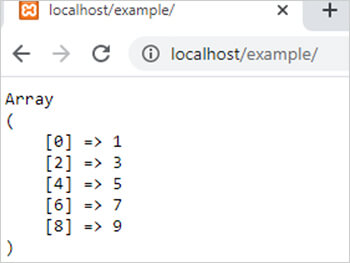array_filter() function in php || PHP Functions

# array_filter() function in php

0 1155

array_filter() function of PHP is used to filter the values of an array with the help of a user define function or callback function. It is an inbuilt function of PHP.

Syntax:

array_filter (\$array_name,\$callback_function_name,\$falg_param);

Here

1. \$array_name is the array which values we want to filter.
2. \$callback function is a user define function.it returns either TRUE or FALSE. If it returns TRUE then the value is added in resultant array. It is not mandatory.
3. \$flag_param is used to specify the arguments in callback function. It has two values. If the value is ARRAY_FILTER_USE_KEY then it takes only keys as arguments but if the value is ARRAY_FILTER_USE_BOTH then it use both keys and values of array to filter the values. It is not mandatory.

Example:

```<?php
/* this example filter odd elements of an array */
function cal_odd(\$arr)
{
if(\$arr%2!=0)
return TRUE;
else
return FALSE;
}
\$arr1= array(1,2,3,4,5,6,7,8,9);
\$result=array_filter(\$arr1,"cal_odd");
echo "<pre>";
print_r(\$result);
?>```

Output:Share: